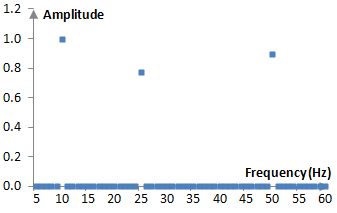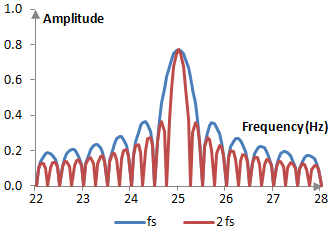# Fourier analysis

Fourier analysis has many applications, but this is topic is an introduction into Fourier analysis as it applies to signal processing.

Suppose that a complex signal x(t) is the sum of simple waves

$$x(t)=\sum_{n=0}^{N-1} A_n \, \cos(2 \pi f_n (t-\tau_n))$$

with different amplitude An, frequency fn, and phase τn. Using trigonometric identities we write

$$x(t)=\sum_{n=0}^{N-1} A_n \, \cos(2 \pi f_n (t-\tau_n))$$ $$=\sum_{n=0}^{N-1}A_n \, \cos(2 \pi f_n \tau_n) \, \cos(2 \pi f_n t)+A_n \, \sin(2 \pi f_n \tau_n) \, \sin(2 \pi f_n t)$$ $$=\sum_{n=0}^{N-1}B_n \, \cos(2 \pi f_n t)+C_n \, \sin(2 \pi f_n t)$$

for some coefficients Bn = An cos(2π fn τn) and Cn = An sin(2π fn τn). Since cos(2π fn t) and sin(2π fn t) are the cosine and sine functions with zero phase, the phase of each simple wave in the signal x(t) is completely determined by the coefficients Bn and Cn and we can work without the phase τn.

## Orthogonal functions and the Fourier series

Sine and cosine functions of different frequencies are orthogonal for carefully chosen intervals and frequencies. If m and n are two different integer frequencies, then

$$\int_0^{2\pi} \cos(m\,t) \, \cos(n\,t) \, dt = \int_0^{2\pi} \frac{1}{2}(\cos((m-n)t)+\cos((m+n)t)) \, dt$$ $$=\frac{1}{2} (-\frac{\sin(2\pi(m-n))-\sin(0)}{m-n}-\frac{\sin(2\pi(m_n))-\sin(0)}{m+n})=0$$

Since m and n are integer and different, the expressions sin(2π (m – n)) and sin(2π (m + n)) evaluate to zero. We can similarly verify that two sine functions are orthogonal and that a sine and a cosine functions are orthogonal in the given interval for integer values of m and n.

The result is not the same for m = n. sin(m t) and cos(m t) are still orthogonal, but sin(m t) and sin(m t) are not, and cos(m t) and cos(m t) are not.

$$\int_0^{2\pi} \cos(m\,t) \, \cos(n\,t) \, dt = \int_0^{2\pi} \frac{1}{2}(\cos((m-n)t)+\cos((m+n)t)) \, dt$$ $$=\frac{1}{2} (2\pi-\frac{\sin(4\pi\,m)-\sin(0)}{m+n})=\pi$$

The sine and cosine functional form that we use above is not our only option. By substituting t with t 2π / T for some interval of time T, we can similarly show that the sine and cosine functions of the form

$$\cos(\frac{2\pi\,n\,t}{T}), \, \sin(\frac{2\pi\,n\,t}{T})$$

are also orthogonal, this time over the interval from 0 to T. If T = 1 and the signal x(t) happens to consist only of integer frequencies fn, then we can say that the signal x(t) is the sum of orthogonal functions.

$$x(t)=\sum_{n=1}^{N} B_n \, \cos(2\pi\,n\,t)+C_n \, \sin(2\pi\,n\,t)$$

The simple waves in x(t) are orthogonal over the interval from 0 to 1, of course, only if x(t) consists of simple waves with integer frequencies.

This is an important result. If x(t) does in fact consist of integer frequencies, then its simple waves are orthogonal in the interval from 0 to 1, and we can compute the magnitudes of the coefficients Bn and Cn.

$$2 \int_0^1 x(t) \, \cos(2\pi\,n\,t) \, dt=2 B_n \, \int_0^1 \cos^2(2\pi\,n\,t) \, dt$$ $$=B_n \int_0^1 (1+\cos(4\pi\,n\,t)) \, dt=B_n(1-\sin(4\pi\,n)+\sin(0))=B_n$$ $$2 \int_0^1 x(t) \, \sin(2\pi\,n\,t) \, dt=C_n$$

## Frequency content of signals

Since, by definition

$$B_n=A_n \, \cos(2\pi\,n\,\tau_n)$$ $$C_n=A_n \, \sin(2\pi\,n\,\tau_n)$$

for some amplitude An and phase τn, then

$$|A_n|=\sqrt{B_n^2+C_N^2}$$ $$2\pi\,n\,\tau_n=\mathrm{atan2}(\frac{C_n}{B_n})$$

We know that An is the amplitude of the n-th simple wave in the signal and 2π n τn is the phase of the n-th simple wave expressed as portions of the wave's cycle.

Thus, if a signal over a properly chosen interval consists of simple waves with integer frequencies, then we can compute the amplitude and phase of each simple frequency in the signal.

In the discrete time case, the above integrals can be approximated as the sum of the sampled function values at, say, N points, multiplied by the sampling interval (1 / N).

$$\frac{2}{N}\sum_{k=0}^{N-1}x(k) \, \cos(\frac{2\pi\,n\,k}{N}) \approx B_n$$ $$\frac{2}{N}\sum_{k=0}^{N-1}x(k) \sin(\frac{2\pi\,n\,k}{N}) \approx C_n$$

The amplitude and phase computations continue to apply.

The amplitude An and phase Φn of a simple wave with integer frequency n in a discrete signal x(k) defined over a period from 0 to 1 are

$$|A_n|=\sqrt{B_n^2+C_n^2}$$ $$\Phi_n=\mathrm{atan2}(\frac{C_n}{B_n})$$

where, for some uniform samples k = 0, 1, …, N

$$B_n=\frac{2}{N} \sum_{k=0}^{N-1} x(k) \, \cos(\frac{2\pi\,n\,k}{N})$$ $$C_n=\frac{2}{N} \sum_{k=0}^{N-1} x(k) \, \sin(\frac{2\pi\,n\,k}{N})$$

One important question remains. We can perform the operations above for complex signals x(k) consisting of simple waves with integer frequencies. Not all complex signals, however, consist of simple waves of integer frequencies. Perhaps not all complex signals consist of simple sine and cosine waves to begin with. We do not know whether practical signals resemble sine and cosine waves in any way. As it turns out, whether or not practical signals are the sums of simple sine and cosine waves is not important.

In 1807 Joseph Fourier shows that any periodic function f(x) with a period of 2π that is integrable over [-π, π] can be approximated with a (potentially infinite) linear sum of sine and cosine waves as follows.

$$f(x) \approx \sum_{n=1}^{N} B_n \, \cos(n\,x)+C_n \, \sin(n\,x)$$

This sum is called the Fourier series of the function f(x). The Fourier series does not always converge and does not always approximate f(x) at every point.

The coefficients Bn and Cn are computed with the integrals

$$B_n=\frac{1}{\pi} \int_{-\pi}^{\pi} f(x) \, \cos(n\,x) \, dx$$ $$C_n=\frac{1}{\pi} \int_{-\pi}^{\pi} f(x) \, \sin(n\,x) \, dx$$

Shifting by π and replacing x with x = 2π t, we can obtain a similar result, namely that a continuous signal x(t) can be approximated with a sum of sine and cosine functions of 2π t over the interval from 0 to 1. We can also produce a similar result in the discrete case.

Thus, whether or not a practical signal is composed of sine and cosine waves, is not important. Within a given interval, we can approximate the signal with a sum of sine and cosine waves similar to the sum we have been using so far.

## Frequency content of an example complex signal

Take a complex signal that is the sum of the simple waves at 10 Hz, 25 Hz, and 50 Hz with amplitude of 1, 0.8 and 0.9 respectively. Suppose that we have the values of the signal sampled with some sampling frequency fs, and nothing else. If we compute the amplitude of various frequencies in the frequency spectrum in this signal using the formulae above over the interval from zero to 1 (from sample 0 to the sampling frequency, thus setting N = fs in these equations), we will obtain the graph in the figure below.We know that simple waves at integer frequencies are orthogonal and so the result is expected. The graph is misleading, however, since we have done nothing about non-integer frequencies. Simple waves at non-integer frequencies are not orthogonal to the simple waves included in the signal for the chosen interval.

The blue line in the figure below shows the frequency content of the signal at non-integer frequencies around 25 Hz. As expected, the content of non-integer frequencies is not necessarily zero. These functions are not orthogonal to the simple waves of the signal in the selected interval. We can, however, make one important observation. Simple waves at non-integer frequencies are orthogonal to simple waves at integer frequencies for properly selected intervals. The simple waves at 5 Hz and 5.5 Hz, for example, are orthogonal in the interval from 0 to 4π, rather than from 0 to 2π as in our previous computations. Thus, we should be able to improve the computation of the frequency content, if we use longer intervals. The red line in the figure below shows the frequency content of the signal around 25 Hz, computed in the interval from zero to twice the sampling frequency. As expected, when doubling the interval, we will also get zero for the frequencies 22.5 Hz, 23.5 Hz, and so on.## Root mean square (RMS) amplitude of a simple wave

The RMS amplitude of a simple wave x(k) = cos(2π f k / fs) with frequency f over the interval from 0 to fs is

$$A_{RMS}=\sqrt{\frac{1}{f_s} \sum_{k=0}^{f_s} x(k)^2}=\sqrt{\frac{1}{f_s} \sum_{k=0}^{f_s} x(k) \, \cos(\frac{2\pi\,f\,k}{f_s})}$$ $$=\sqrt{\frac{B_n}{2}}=\sqrt{\frac{1}{2}} \approx 0.707107$$

This is so because a simple cosine wave has a Fourier series expansion that is itself and Bn = 1. The result is the same for a simple sine wave.

### Filtered HTML

• Freelinking helps you easily create HTML links. Links take the form of [[indicator:target|Title]]. By default (no indicator): Click to view a local node.